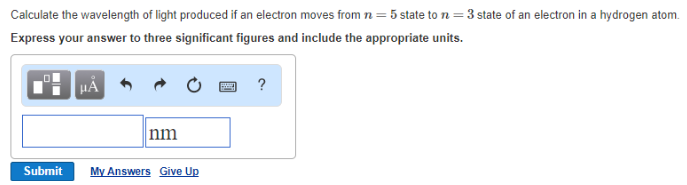# Problem: Calculate the wavelength of light produced if an electron moves from n = 5 state to n = 3 state of an electron in a hydrogen atom.Express your answer to three significant figures and include the appropriate units.

###### FREE Expert Solution
86% (212 ratings)###### Problem Details

Calculate the wavelength of light produced if an electron moves from n = 5 state to n = 3 state of an electron in a hydrogen atom.

Express your answer to three significant figures and include the appropriate units.Frequently Asked Questions

What scientific concept do you need to know in order to solve this problem?

Our tutors have indicated that to solve this problem you will need to apply the Bohr Equation concept. You can view video lessons to learn Bohr Equation. Or if you need more Bohr Equation practice, you can also practice Bohr Equation practice problems.

What professor is this problem relevant for?

Based on our data, we think this problem is relevant for Professor Drolet's class at OREGONSTATE.